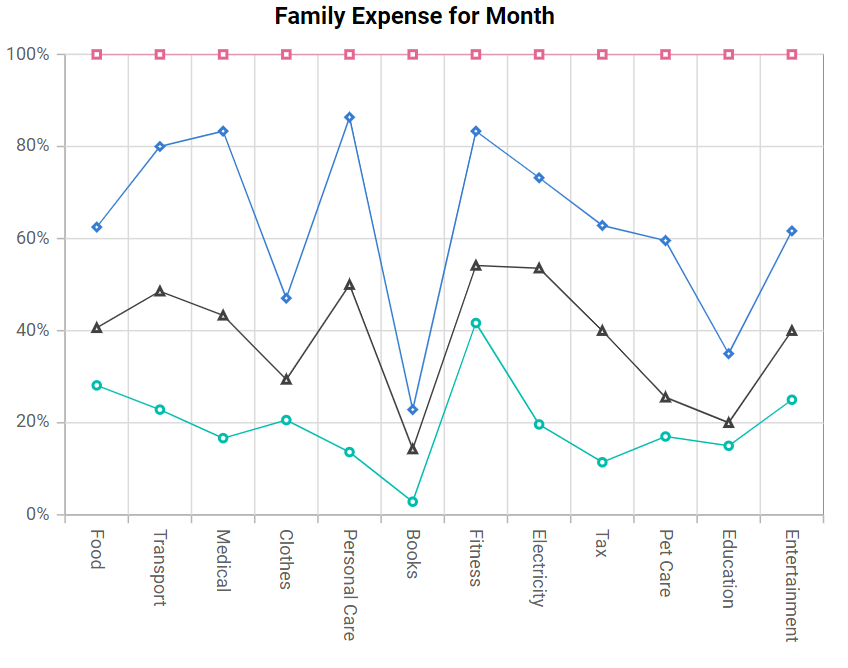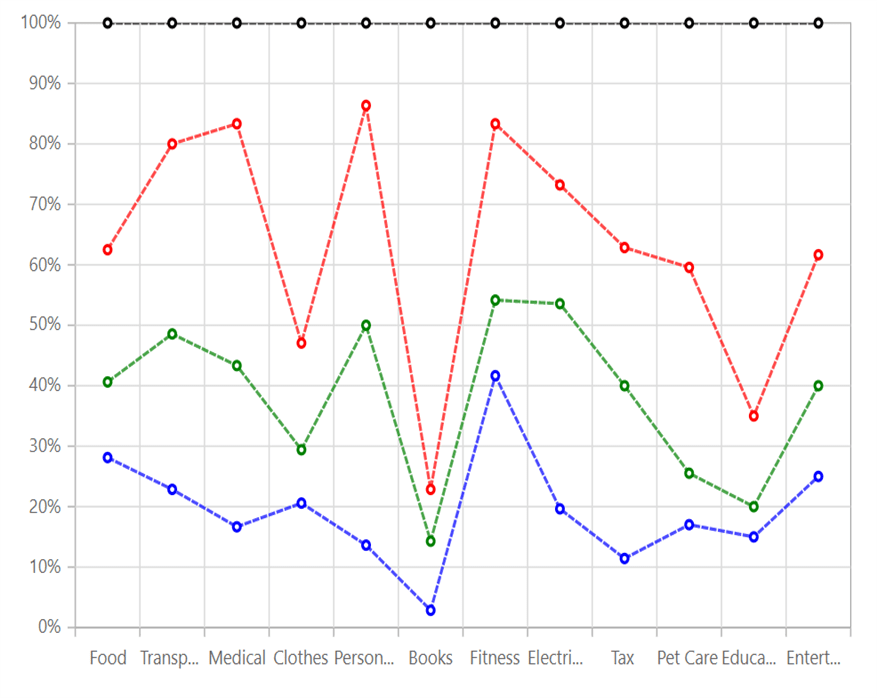# 100% Stacked Line in Blazor Charts Component

11 Aug 20239 minutes to read

## 100% Stacked Line

100% Stacked Line Chart displays multiple series of data as stacked lines, ensuring that the cumulative proportion of each stacked element always totals 100%. Hence, the y-axis will always be rendered with the range 0–100%. To render a 100% Stacked Line series, set the series Type as StackingLine100.

``````@using Syncfusion.Blazor.Charts

<SfChart Title="Family Expense for Month">
<ChartPrimaryXAxis ValueType="Syncfusion.Blazor.Charts.ValueType.Category">
</ChartPrimaryXAxis>

<ChartSeriesCollection>
<ChartSeries XName="X" Width="2" DashArray="5,1" DataSource="@ExpenseReports"
YName="Y" Type="ChartSeriesType.StackingLine100">
<ChartMarker Visible="true">
</ChartMarker>
</ChartSeries>
<ChartSeries XName="X" Width="2" DashArray="5,1" DataSource="@ExpenseReports"
YName="Y1" Type="ChartSeriesType.StackingLine100">
<ChartMarker Visible="true">
</ChartMarker>
</ChartSeries>
<ChartSeries XName="X" Width="2" DashArray="5,1" DataSource="@ExpenseReports"
YName="Y2" Type="ChartSeriesType.StackingLine100">
<ChartMarker Visible="true">
</ChartMarker>
</ChartSeries>
<ChartSeries XName="X" Width="2" DashArray="5,1" DataSource="@ExpenseReports"
YName="Y3" Type="ChartSeriesType.StackingLine100">
<ChartMarker Visible="true">
</ChartMarker>
</ChartSeries>
</ChartSeriesCollection>
</SfChart>

@code{
public class ChartData
{
public string X { get; set; }
public double Y { get; set; }
public double Y1 { get; set; }
public double Y2 { get; set; }
public double Y3 { get; set; }
}

public List<ChartData> ExpenseReports = new List<ChartData>
{
new ChartData { X = "Food" , Y = 90, Y1 = 40 , Y2= 70, Y3= 120},
new ChartData { X = "Transport", Y = 80, Y1 = 90, Y2= 110, Y3= 70 },
new ChartData { X = "Medical",Y = 50, Y1 = 80, Y2= 120, Y3= 50 },
new ChartData { X = "Clothes",Y = 70, Y1 = 30, Y2= 60, Y3= 180 },
new ChartData { X = "Personal Care", Y = 30, Y1 = 80, Y2= 80, Y3= 30 },
new ChartData { X = "Books", Y = 10, Y1 = 40, Y2= 30, Y3= 270},
new ChartData { X = "Fitness",Y = 100, Y1 = 30, Y2= 70, Y3= 40 },
new ChartData { X = "Electricity", Y = 55, Y1 = 95, Y2= 55, Y3= 75},
new ChartData { X = "Tax", Y = 20, Y1 = 50, Y2= 40, Y3= 65 },
new ChartData { X = "Pet Care", Y = 40, Y1 = 20, Y2= 80, Y3= 95 },
new ChartData { X = "Education", Y = 45, Y1 = 15, Y2= 45, Y3= 195 },
new ChartData { X = "Entertainment", Y = 75, Y1 = 45, Y2= 65, Y3= 115 }
};
}``````NOTE

Refer to our Blazor 100% Stacked Line Chart feature tour page to know about its other groundbreaking feature representations. Explore our Blazor 100% Stacked Line Chart Example to know how to render and configure the 100% Stacked Line type chart.

## Series customization

The following properties can be used to customize the 100% Stacked Line series.

• Fill – Specifies the color of the series.
• Opacity – Specifies the opacity of Fill.
• Width – Specifies the width of the line stroke.
• DashArray – Specifies the dashes of line stroke.
``````@using Syncfusion.Blazor.Charts

<SfChart Title="Family Expense for Month">
<ChartPrimaryXAxis ValueType="Syncfusion.Blazor.Charts.ValueType.Category">
</ChartPrimaryXAxis>

<ChartSeriesCollection>
<ChartSeries XName="X" Width="2" DashArray="5,1" DataSource="@ExpenseReports"
YName="Y" Fill="blue" Opacity="0.7" Type="ChartSeriesType.StackingLine100">
<ChartMarker Visible="true">
</ChartMarker>
</ChartSeries>
<ChartSeries XName="X" Width="2" DashArray="5,1" DataSource="@ExpenseReports"
YName="Y1" Fill="green" Opacity="0.7" Type="ChartSeriesType.StackingLine100">
<ChartMarker Visible="true">
</ChartMarker>
</ChartSeries>
<ChartSeries XName="X" Width="2" DashArray="5,1" DataSource="@ExpenseReports"
YName="Y2" Fill="red" Opacity="0.7" Type="ChartSeriesType.StackingLine100">
<ChartMarker Visible="true">
</ChartMarker>
</ChartSeries>
<ChartSeries XName="X" Width="2" DashArray="5,1" DataSource="@ExpenseReports"
YName="Y3" Fill="black" Opacity="0.7" Type="ChartSeriesType.StackingLine100">
<ChartMarker Visible="true">
</ChartMarker>
</ChartSeries>
</ChartSeriesCollection>
</SfChart>

@code{
public class ChartData
{
public string X { get; set; }
public double Y { get; set; }
public double Y1 { get; set; }
public double Y2 { get; set; }
public double Y3 { get; set; }
}

public List<ChartData> ExpenseReports = new List<ChartData>
{
new ChartData { X = "Food" , Y = 90, Y1 = 40 , Y2= 70, Y3= 120},
new ChartData { X = "Transport", Y = 80, Y1 = 90, Y2= 110, Y3= 70 },
new ChartData { X = "Medical",Y = 50, Y1 = 80, Y2= 120, Y3= 50 },
new ChartData { X = "Clothes",Y = 70, Y1 = 30, Y2= 60, Y3= 180 },
new ChartData { X = "Personal Care", Y = 30, Y1 = 80, Y2= 80, Y3= 30 },
new ChartData { X = "Books", Y = 10, Y1 = 40, Y2= 30, Y3= 270},
new ChartData { X = "Fitness",Y = 100, Y1 = 30, Y2= 70, Y3= 40 },
new ChartData { X = "Electricity", Y = 55, Y1 = 95, Y2= 55, Y3= 75},
new ChartData { X = "Tax", Y = 20, Y1 = 50, Y2= 40, Y3= 65 },
new ChartData { X = "Pet Care", Y = 40, Y1 = 20, Y2= 80, Y3= 95 },
new ChartData { X = "Education", Y = 45, Y1 = 15, Y2= 45, Y3= 195 },
new ChartData { X = "Entertainment", Y = 75, Y1 = 45, Y2= 65, Y3= 115 }
};
}``````NOTE

Refer to our Blazor Charts feature tour page for its groundbreaking feature representations and also explore our Blazor Chart Example to know various chart types and how to represent time-dependent data, showing trends at equal intervals.